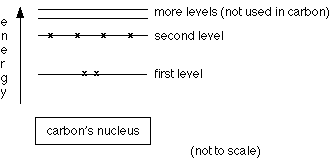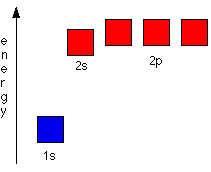# Electronic Structure and Orbitals

$$\newcommand{\vecs}{\overset { \rightharpoonup} {\mathbf{#1}} }$$ $$\newcommand{\vecd}{\overset{-\!-\!\rightharpoonup}{\vphantom{a}\smash {#1}}}$$$$\newcommand{\id}{\mathrm{id}}$$ $$\newcommand{\Span}{\mathrm{span}}$$ $$\newcommand{\kernel}{\mathrm{null}\,}$$ $$\newcommand{\range}{\mathrm{range}\,}$$ $$\newcommand{\RealPart}{\mathrm{Re}}$$ $$\newcommand{\ImaginaryPart}{\mathrm{Im}}$$ $$\newcommand{\Argument}{\mathrm{Arg}}$$ $$\newcommand{\norm}{\| #1 \|}$$ $$\newcommand{\inner}{\langle #1, #2 \rangle}$$ $$\newcommand{\Span}{\mathrm{span}}$$ $$\newcommand{\id}{\mathrm{id}}$$ $$\newcommand{\Span}{\mathrm{span}}$$ $$\newcommand{\kernel}{\mathrm{null}\,}$$ $$\newcommand{\range}{\mathrm{range}\,}$$ $$\newcommand{\RealPart}{\mathrm{Re}}$$ $$\newcommand{\ImaginaryPart}{\mathrm{Im}}$$ $$\newcommand{\Argument}{\mathrm{Arg}}$$ $$\newcommand{\norm}{\| #1 \|}$$ $$\newcommand{\inner}{\langle #1, #2 \rangle}$$ $$\newcommand{\Span}{\mathrm{span}}$$$$\newcommand{\AA}{\unicode[.8,0]{x212B}}$$

In any introductory chemistry course you will have come across the electronic structures of hydrogen and carbon drawn as:The circles show energy levels - representing increasing distances from the nucleus. You could straighten the circles out and draw the electronic structure as a simple energy diagram.## Atomic Orbitals

Orbits and orbitals sound similar, but they have quite different meanings. It is essential that you understand the difference between them. To plot a path for something you need to know exactly where the object is and be able to work out exactly where it's going to be an instant later. You can't do this for electrons.

The Heisenberg Uncertainty Principle says that you cannot know with certainty both where an electron is and where it's going next. That makes it impossible to plot an orbit for an electron around a nucleus. Is this a big problem? No. If something is impossible, you have to accept it and find a way around it.

### Hydrogen's electron - the 1s orbital

Suppose you had a single hydrogen atom and at a particular instant plotted the position of the one electron. Soon afterwards, you do the same thing, and find that it is in a new position. You have no idea how it got from the first place to the second. You keep on doing this over and over again, and gradually build up a sort of 3D map of the places that the electron is likely to be found.

In the hydrogen case, the electron can be found anywhere within a spherical space surrounding the nucleus. The diagram shows a cross-section through this spherical space. 95% of the time (or any other percentage you choose), the electron will be found within a fairly easily defined region of space quite close to the nucleus. Such a region of space is called an orbital. You can think of an orbital as being the region of space in which the electron lives.What is the electron doing in the orbital? We don't know, we can't know, and so we just ignore the problem! All you can say is that if an electron is in a particular orbital it will have a particular definable energy.

Each orbital has a name. The orbital occupied by the hydrogen electron is called a 1s orbital. The "1" represents the fact that the orbital is in the energy level closest to the nucleus. The "s" tells you about the shape of the orbital. s orbitals are spherically symmetric around the nucleus - in each case, like a hollow ball made of rather chunky material with the nucleus at its center.

The orbital on the left is a 2s orbital. This is similar to a 1s orbital except that the region where there is the greatest chance of finding the electron is further from the nucleus - this is an orbital at the second energy level. If you look carefully, you will notice that there is another region of slightly higher electron density (where the dots are thicker) nearer the nucleus. ("Electron density" is another way of talking about how likely you are to find an electron at a particular place.)

2s (and 3s, 4s, etc) electrons spend some of their time closer to the nucleus than you might expect. The effect of this is to slightly reduce the energy of electrons in s orbitals. The nearer the nucleus the electrons get, the lower their energy. 3s, 4s (etc) orbitals get progressively further from the nucleus.

### p orbitalsNot all electrons inhabit s orbitals (in fact, very few electrons live in s orbitals). At the first energy level, the only orbital available to electrons is the 1s orbital, but at the second level, as well as a 2s orbital, there are also orbitals called 2p orbitals. A p orbital is rather like 2 identical balloons tied together at the nucleus. The diagram on the right is a cross-section through that 3-dimensional region of space. Once again, the orbital shows where there is a 95% chance of finding a particular electron.

Unlike an s orbital, a p orbital points in a particular direction - the one drawn points up and down the page. At any one energy level it is possible to have three absolutely equivalent p orbitals pointing mutually at right angles to each other. These are arbitrarily given the symbols px, py and pz. This is simply for convenience - what you might think of as the x, y or z direction changes constantly as the atom tumbles in space.The p orbitals at the second energy level are called 2px, 2py and 2pz. There are similar orbitals at subsequent levels - 3px, 3py, 3pz, 4px, 4py, 4pz and so on. All levels except for the first level have p orbitals. At the higher levels the lobes get more elongated, with the most likely place to find the electron more distant from the nucleus.

## Fitting electrons into orbitals

Because for the moment we are only interested in the electronic structures of hydrogen and carbon, we do not need to concern ourselves with what happens beyond the second energy level. Remember:

• At the first level there is only one orbital - the $$1s$$ orbital.
• At the second level there are four orbitals - the $$2s$$, $$2p_x$$, $$2p_y$$ and $$2p_z$$ or bitals.
• Each orbital can hold either 1 or 2 electrons, but no more.

## "Electrons-in-boxes"

Orbitals can be represented as boxes with the electrons in them shown as arrows. Often an up-arrow and a down-arrow are used to show that the electrons are in some way different. A 1s orbital holding 2 electrons would be drawn as shown on the right, but it can be written even more quickly as 1s2. This is read as "one s two" - not as "one s squared". You mustn't confuse the two numbers in this notation:## The order of filling orbitals

Electrons fill low energy orbitals (closer to the nucleus) before they fill higher energy ones. Where there is a choice between orbitals of equal energy, they fill the orbitals singly as far as possible. The diagram (not to scale) summarizes the energies of the various orbitals in the first and second levels.Notice that the 2s orbital has a slightly lower energy than the 2p orbitals. That means that the 2s orbital will fill with electrons before the 2p orbitals. All the 2p orbitals have exactly the same energy.

Example 1: The electronic structure of hydrogen
Hydrogen only has one electron and that will go into the orbital with the lowest energy - the 1s orbital. Hydrogen has an electronic structure of 1s1. We have already described this orbital earlier.
Example 2: The electronic structure of carbon

Carbon has six electrons. Two of them will be found in the 1s orbital close to the nucleus. The next two will go into the 2s orbital. The remaining ones will be in two separate 2p orbitals. This is because the p orbitals all have the same energy and the electrons prefer to be on their own if that's the case.The electronic structure of carbon is normally written 1s22s22px12py10

## Contributor

Jim Clark (Chemguide.co.uk)

.

This page titled Electronic Structure and Orbitals is shared under a CC BY-NC 4.0 license and was authored, remixed, and/or curated by Jim Clark.